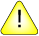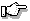This version of this document is no longer maintained. For the latest documentation, see http://www.qnx.com/developers/docs.

scalb(), scalbf()

Synopsis:

#include <math.h>

double scalb( double x,
double n );

float scalbf( float x,
float n );

Arguments:

x
The floating point number that you want to multiply by the exponent.
n
The exponent to apply to the radix of the machine's floating-point arithmetic.

Library:

libm

Use the -l m option to qcc to link against this library.

Description:

These functions compute x * rn, where r is the radix of the machine's floating point arithmetic and n is a finite number. When r is 2, scalb() is equivalent to ldexp().We recommend that you use scalbn() because it computes by manipulating exponents, instead of using mock multiplications or additions. The scalbf() function is based on an ANSI draft; for more portable code, use scalbnf() instead.

Returns:

x * rnIf an error occurs, these functions return 0, but this is also a valid mathematical result. If you want to check for errors, set errno to 0, call the function, and then check errno again. These functions don't change errno if no errors occurred.

Examples:

#include <stdio.h>
#include <errno.h>
#include <inttypes.h>
#include <math.h>
#include <fpstatus.h>

int main(int argc, char** argv)
{
double a, b, c, d;

a = 10;
b = 2;
c = scalb(a, b);
d = sqrt(c/a);
printf("Radix of machines fp arithmetic is %f \n", d);
printf("So %f = %f * (%f ^ %f) \n", c, a, d, b);

return(0);
}

produces the output:

Radix of machines fp arithmetic is 2.000000
So 40.000000 = 10.000000 * (2.000000 ^ 2.000000)

Classification:

scalb() is POSIX 1003.1 XSI; scalbf() is ANSI (draft)

Safety:
Cancellation point No
Interrupt handler No
Signal handler No